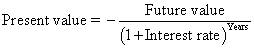##### Managerial Accounting For Dummies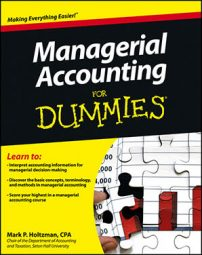Managerial accountants compute and provide information within a company. Managerial accounting information is numeric, calculated using certain formulas. The following list summarizes some of the most important formulas in managerial accounting.

## The accounting equation

The accounting equation equates assets with liabilities and owners’ equity:

Assets = Liability + Owners' Equity

Assets are things owned by the company — such as cash, inventory, and equipment — that will provide some future benefit. Liabilities entail future sacrifices that the company must make, such as paying bills or other kinds of debts. Owners’ equity represents the portion of the company that actually belongs to the owner.

A basic rule of accounting is that the accounting equation must always balance. If assets exceed the sum of liabilities and owners’ equity, then the company holds things that don’t belong to anyone. If the sum of liabilities and owners’ equity exceeds assets, then owners and creditors lay claim to things that don’t exist.

## Net income

Net income is called the bottom line because in many ways it’s the sum total of accountants’ work. To calculate net income, subtract expenses from revenues:

Revenues – Expenses = Net income

Revenues are inflows and other kinds of sales to customers. Expenses are costs associated with making sales. Accountants also sometimes need to add gains or subtract losses in net income; these gains and losses come from miscellaneous events that affect stockholder value, such as selling equipment at a gain or getting your factory destroyed by a mutated prehistoric survivor of the dinosaurs.

## Cost of goods sold

For manufacturers and retailers, cost of goods sold measures how much the company paid — or will need to pay — for inventory items sold.

To compute a retailer’s cost of goods sold, use the following formula:

Beginning + Inputs = Outputs
Cost of beginning inventory + Cost of purchases – Cost of ending inventory = Costs of goods sold

Here, a retailer’s inputs are the cost of the purchases it makes. The outputs are the goods that were sold (recorded at cost, of course).

## Contribution margin

Contribution margin measures how selling one item, or a group of items, increases net income. To calculate contribution margin, subtract variable costs from sales:

Total sales – Total variable cost = Total contribution margin

Contribution margin helps managers by explaining how decisions will impact income. Should you prepare a special order with a contribution margin of \$100,000? Yes, because it will increase net income by \$100,000. Should you prepare another special order with a contribution margin of negative \$50,000? No, because it will decrease net income.

To compute contribution margin per unit, divide the total contribution margin by the number of units sold. Alternatively, you can calculate sales price less variable cost per unit:

Sales price – Variable cost per unit = Contribution margin per unit

To compute contribution margin ratio, divide contribution margin by sales, either in total or per unit: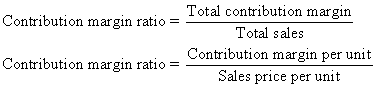## Cost-volume profit analysis

Cost-volume-profit (CVP) analysis helps you understand how changes in volume affect costs and net income. If you know sales price, variable cost per unit, volume, and fixed costs, this formula will predict your net income:

Net income = (Sales price – Variable cost per unit)(Volume) – Fixed costs

First, understand where this formula comes from. Consider how production volume affects total costs:

Total cost = (Variable cost per unit x Volume) + Fixed costs

Variable cost per unit is the additional cost of producing a single unit. Volume is the number of units produced. Fixed cost is the total fixed cost for the period. Net income is just the difference between total sales and total cost:

Net income = (Sales price x Volume) – Total cost

Combining these two equations gives you the super-useful formula for understanding how volume affects profits: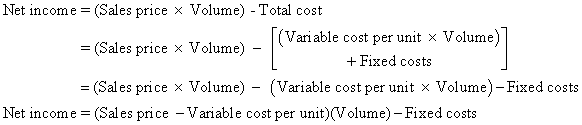Not coincidentally, a critical part of this formula equals contribution margin — remember that sales price less variable cost per unit equals contribution margin per unit:

Sales price – Variable cost per unit = Contribution margin per unit

This formula lets you further simplify the CVP formula:

Net income = (Contribution margin x Volume) – Fixed costs

## Break-even analysis

Break-even analysis helps you determine how much you need to sell in order to break even — that is, to earn no net loss or profit. To figure out the break-even point, use this formula: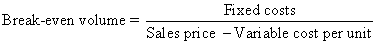Perhaps you recognize contribution margin in the denominator (Sales price – Variable cost per unit), allowing you to further simplify this formula: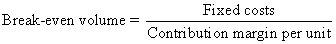To figure out the number of units needed to break even, just divide total fixed costs by contribution margin per unit.

## Price variance

Price variance tells you how an unexpected change in the cost of direct materials affects total cost. Use this formula to compute price variance:

Price variance = (Standard price – Actual price) x Actual quantity

Standard price is the amount you originally expected to pay, per unit, of direct materials. Actual price is the real price you paid, per unit, for direct materials. The actual quantity is the number of units purchased and used in production.

Although the price variance formula focuses on the direct materials variance, you can easily adapt it to figure out the direct labor variance. To do so, replace standard price with the standard cost (per hour) of direct labor. Replace actual price with the actual cost (per hour) of direct labor. Then replace the actual quantity with the actual number of hours worked.

## Quantity variance

The direct materials quantity variance measures how using too much or too little in direct materials affects total costs. Stinginess in using direct materials should decrease your costs. However, wasting direct materials should increase costs. Here’s the formula:

Quantity variance = Standard price x (Standard quantity – Actual quantity)

Remember that standard price is how much you originally expected to pay, per unit, of direct materials. Standard quantity is the number of units of direct materials that you expected to use. Actual quantity is the number of units of direct materials that you actually used in production.

## Future value

Future value measures how much a present cash flow will be worth in the future. For example, if you put \$1,000 into the bank today, earning 6-percent interest a year, how much will you have ten years from now?

To solve these problems, many students use tables printed in textbooks or financial calculators. You can also solve these problems using the time value of money formula:

Future value = –Present value x (1 + interest rate)Years

Present value measures how much money you receive or pay now. Make this figure positive if you’re receiving the money and negative if you’re paying the money out. Future value is how much you can expect to receive or pay in the future (again, positive for incoming cash, negative for outgoing cash).

The interest rate should be put in as the annual interest rate (rather than daily, monthly, or quarterly). The number of years is for the period of time between the date of the present value and the date of the future value, in years.

Therefore, if present value equals –\$1,000, the interest rate is 6 percent, and the number of years is ten years.

Future value = –(–\$1,000) x (1 + 0.06)10
= \$1,000 x (1.06) 10
= \$1,000 x 1.791
= \$1.791

The future value indicates that, if you put \$1,000 away now, earning 6 percent, you can expect to receive \$1,791 at the end of ten years.

## Present value

Present value uses the same formula as future value.

Future value = –Present value x (1 + interest rate)Years

Here’s an example of how you can use this formula to compute the present value of a cash flow. Suppose that, four years from now, you want to have \$5,000 (that’s the future value). How much should you put into the bank today, earning 5-percent interest?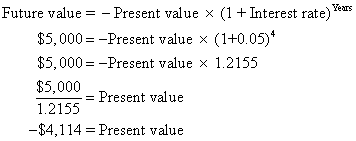So if you put \$4,114 into the bank today, earning 5-percent interest, then in four years you should have \$5,000 to take out.

Here’s a version of the formula to more directly compute present value: